# ParameterConfidenceInterval

Object containing confidence interval results for estimated parameters

## Description

The `ParameterConfidenceInterval` object contains confidence interval results for the estimated parameters computed using `sbioparameterci`.

## Creation

### Syntax

``ci = sbioparameterci(fitResults)``
``ci = sbioparameterci(fitResults,Name,Value)``

### Description

example

````ci = sbioparameterci(fitResults)` computes 95% confidence intervals for the estimated parameters from `fitResults`, a `NLINResults object` or `OptimResults object` returned by `sbiofit`. `ci` is a `ParameterConfidenceInterval` object that contains the computed confidence intervals.```

example

````ci = sbioparameterci(fitResults,Name,Value)` uses additional options specified by one or more `Name,Value` pair arguments.```

### Input Arguments

expand all

Parameter estimation results from `sbiofit`, specified as an `NLINResults object`, `OptimResults object`, or a vector of objects for unpooled fits that were returned from the same `sbiofit` call.

#### Name-Value Pair Arguments

Confidence level, `(1-Alpha) * 100%`, specified as the comma-separated pair consisting of `'Alpha'` and a positive scalar between 0 and 1. The default value is `0.05`, meaning a 95% confidence interval is computed.

Example: `'Alpha',0.01`

Confidence interval type, specified as the comma-separated pair consisting of `'Type'` and a character vector. The valid choices are:

• `'gaussian'`– Use the Gaussian approximation of the distribution of parameter estimates.

• `'profileLikelihood'`– Compute the profile likelihood intervals. This type is not supported for parameter estimates from hierarchical models, that is, estimated results from fitting different categories (such as Age or Sex). In other words, if you set the `CategoryVariableName` property of the `EstimatedInfo object` in your original fit, then the fit results are hierarchical, therefore, you cannot compute the `profileLikelihood` confidence intervals on the results. For details, see Profile Likelihood Confidence Interval Calculation.

• `'bootstrap'`– Compute confidence intervals using the bootstrap method.

Example: `'Type','bootstrap'`

Tolerance for the profile likelihood and bootstrap confidence interval computations, specified as the comma-separated pair consisting of `'Tolerance'` and a positive scalar.

The profile likelihood method uses this value as a termination tolerance. For details, see Profile Likelihood Confidence Interval Calculation.

The bootstrap method uses this value to determine whether a confidence interval is constrained by bounds specified in the original fit. For details, see Bootstrap Confidence Interval Calculation.

Example: `'Tolerance',1e-6`

Maximum step size used for computing profile likelihood curves, specified as the comma-separated pair consisting of `'MaxStepSize'` and a positive scalar, `[]`, or cell array. By default, this argument is set to `0.1`. If you set it to `[]`, then the maximum step size is set to 10% of the width of the Gaussian approximation of the confidence interval, if it exists. You can specify a maximum step size (or `[]`) for each estimated parameter using a cell array.

Example: `'MaxStepSize',0.5`

Number of samples for bootstrapping, specified as the comma-separated pair consisting of `'NumSamples'` and a positive integer. This number defines the number of fits that are performed during the confidence interval computation to generate bootstrap samples. The smaller the number is, the faster the computation of the confidence intervals becomes, at the cost of decreased accuracy.

Example: `'NumSamples',500`

Level of display returned to the command line, specified as the comma-separated pair consisting of `'Display'` and a character vector. `'off'` (default) or `'none'` displays no output. `'final'` displays a message when a computation finishes. `'iter'` displays output at each iteration.

Example: `'Display','final'`

Logical flag to compute confidence intervals in parallel, specified as the comma-separated pair consisting of `'UseParallel'` and `true` or `false`. By default, the parallel options in the original fit are used. If this argument is set to `true` and Parallel Computing Toolbox™ is available, the parallel options in the original fit are ignored, and confidence intervals are computed in parallel.

For the Gaussian confidence intervals:

• If the input `fitResults` is a vector of results objects, then the computation of confidence intervals for each object is performed in parallel. The Gaussian confidence intervals are quick to compute. So, it might be more beneficial to parallelize the original fit (`sbiofit`) and not set `UseParallel` to true for `sbioparameterci`.

For the Profile Likelihood confidence intervals:

• If the number of results objects in the input `fitResults` vector is greater than the number of estimated parameters, then the computation of confidence intervals for each object is performed in parallel.

• Otherwise, the confidence intervals for all estimated parameters within one results object are computed in parallel before the function moves on to the next results object.

For the Bootstrap confidence intervals:

• The function forwards the `UseParallel` flag to `bootci`. There is no parallelization over the input vector of results objects.

### Note

If you have a global stream for random number generation with several substreams to compute in parallel in a reproducible fashion, `sbioparameterci` first checks to see if the number of workers is same as the number of substreams. If so, `sbioparameterci` sets `UseSubstreams` to `true` in the `statset` option and passes it to `bootci`. Otherwise, the substreams are ignored by default.

Example: `'UseParallel',true`

## Properties

expand all

Confidence interval type, specified as `'gaussian'`, `'profileLikelihood'`, or `'bootstrap'`.

Example: `'bootstrap'`

Confidence level, `(1-Alpha) * 100%`, specified as a positive scalar between 0 and 1.

Example: `0.01`

Original group names from the data used for fitting the model, specified as a cell array of character vectors. Each cell contains the name of a group.

Example: `{'1'}{'2'}{'3'}`

Confidence interval results, specified as a table. The table contains the following columns.

Column NameDescription
NameName of the estimated parameter
EstimateEstimated parameter value
BoundsLower and upper parameter bounds (if defined in the original fit)
GroupGroup name (if available)
CategoryVariableName Name of category (if defined in the original fit)
CategoryValueValue of the category variable specified by CategoryVariableName
ConfidenceIntervalConfidence interval values
StatusConfidence interval estimation status, specified as one of the following categorical values: `success`, `constrained`, `estimable`, ```not estimable``` (for details, see Parameter Confidence Interval Estimation Status)

Exit flags returned during the calculation of `bootstrap` confidence intervals only, specified as a vector of integers. Each integer is an exit flag returned by the estimation function (except `nlinfit`) used to fit parameters during bootstrapping. The same estimation function used in the original fit is used for bootstrapping.

Each flag indicates the success or failure status of the fitting performed to create a bootstrap sample. Refer to the reference page of the corresponding estimation function for the meaning of the exit flag.

If the estimation function does not return an exit flag, `ExitFlags` is set to `[]`. For the `gaussian` and `profileLikelihood` confidence intervals, `ExitFlags` is not supported and is always set to `[]`.

## Object Functions

 `ci2table` Return summary table of confidence interval results `plot` Plot parameter confidence interval results

## Examples

collapse all

Load the sample data to fit. The data is stored as a table with variables ID , Time , CentralConc , and PeripheralConc. This synthetic data represents the time course of plasma concentrations measured at eight different time points for both central and peripheral compartments after an infusion dose for three individuals.

```clear all load data10_32R.mat gData = groupedData(data); gData.Properties.VariableUnits = {'','hour','milligram/liter','milligram/liter'}; sbiotrellis(gData,'ID','Time',{'CentralConc','PeripheralConc'},'Marker','+',... 'LineStyle','none'); ```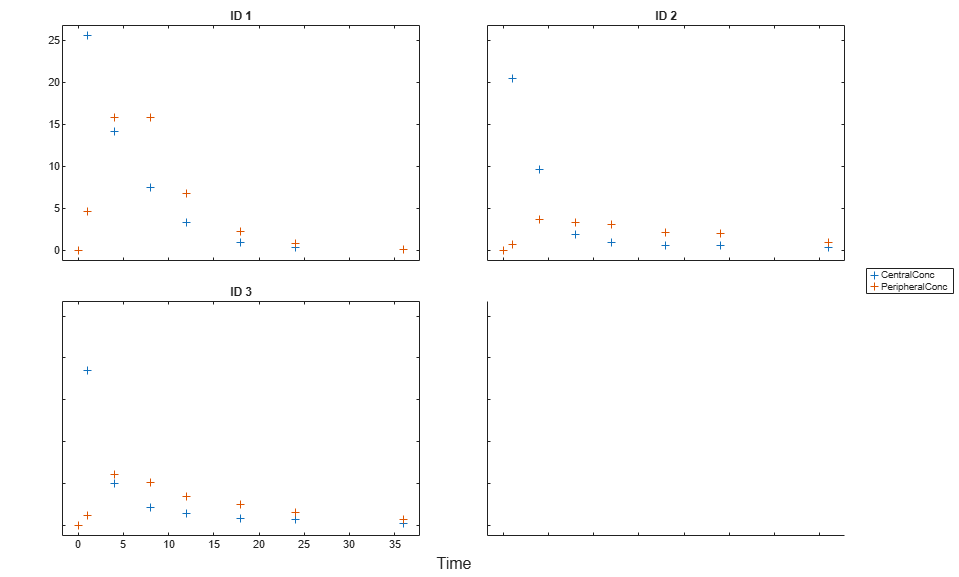Create Model

Create a two-compartment model.

```pkmd = PKModelDesign; pkc1 = addCompartment(pkmd,'Central'); pkc1.DosingType = 'Infusion'; pkc1.EliminationType = 'linear-clearance'; pkc1.HasResponseVariable = true; pkc2 = addCompartment(pkmd,'Peripheral'); model = construct(pkmd); configset = getconfigset(model); configset.CompileOptions.UnitConversion = true; ```

Define Dosing

Define the infusion dose.

```dose = sbiodose('dose','TargetName','Drug_Central'); dose.StartTime = 0; dose.Amount = 100; dose.Rate = 50; dose.AmountUnits = 'milligram'; dose.TimeUnits = 'hour'; dose.RateUnits = 'milligram/hour'; ```

Define Parameters

Define the parameters to estimate. Set the parameter bounds for each parameter. In addition to these explicit bounds, the parameter transformations (such as log, logit, or probit) impose implicit bounds.

```responseMap = {'Drug_Central = CentralConc','Drug_Peripheral = PeripheralConc'}; paramsToEstimate = {'log(Central)','log(Peripheral)','Q12','Cl_Central'}; estimatedParam = estimatedInfo(paramsToEstimate,... 'InitialValue',[1 1 1 1],... 'Bounds',[0.1 3;0.1 10;0 10;0.1 2]); ```

Fit Model

Perform an unpooled fit, that is, one set of estimated parameters for each patient.

```unpooledFit = sbiofit(model,gData,responseMap,estimatedParam,dose,'Pooled',false); ```

Perform a pooled fit, that is, one set of estimated parameters for all patients.

```pooledFit = sbiofit(model,gData,responseMap,estimatedParam,dose,'Pooled',true); ```

Compute Confidence Intervals for Estimated Parameters

Compute 95% confidence intervals for each estimated parameter in the unpooled fit.

```ciParamUnpooled = sbioparameterci(unpooledFit); ```

Display Results

Display the confidence intervals in a table format. For details about the meaning of each estimation status, see Parameter Confidence Interval Estimation Status.

```ci2table(ciParamUnpooled) ```
```ans = 12x7 table Group Name Estimate ConfidenceInterval Type Alpha Status _____ ______________ ________ __________________ ________ _____ ___________ 1 {'Central' } 1.422 1.1533 1.6906 Gaussian 0.05 estimable 1 {'Peripheral'} 1.5629 0.83143 2.3551 Gaussian 0.05 constrained 1 {'Q12' } 0.47159 0.20093 0.80247 Gaussian 0.05 constrained 1 {'Cl_Central'} 0.52898 0.44842 0.60955 Gaussian 0.05 estimable 2 {'Central' } 1.8322 1.7893 1.8751 Gaussian 0.05 success 2 {'Peripheral'} 5.3368 3.9133 6.7602 Gaussian 0.05 success 2 {'Q12' } 0.27641 0.2093 0.34351 Gaussian 0.05 success 2 {'Cl_Central'} 0.86034 0.80313 0.91755 Gaussian 0.05 success 3 {'Central' } 1.6657 1.5818 1.7497 Gaussian 0.05 success 3 {'Peripheral'} 5.5632 4.7557 6.3708 Gaussian 0.05 success 3 {'Q12' } 0.78361 0.65581 0.91142 Gaussian 0.05 success 3 {'Cl_Central'} 1.0233 0.96375 1.0828 Gaussian 0.05 success ```

Plot the confidence intervals. If the estimation status of a confidence interval is `success`, it is plotted in blue (the first default color). Otherwise, it is plotted in red (the second default color), which indicates that further investigation into the fitted parameters may be required. If the confidence interval is `not estimable`, then the function plots a red line with a centered cross. If there are any transformed parameters with estimated values 0 (for the log transform) and 1 or 0 (for the probit or logit transform), then no confidence intervals are plotted for those parameter estimates. To see the color order, type `get(groot,'defaultAxesColorOrder')`.

Groups are displayed from left to right in the same order that they appear in the `GroupNames` property of the object, which is used to label the x-axis. The y-labels are the transformed parameter names.

```plot(ciParamUnpooled) ```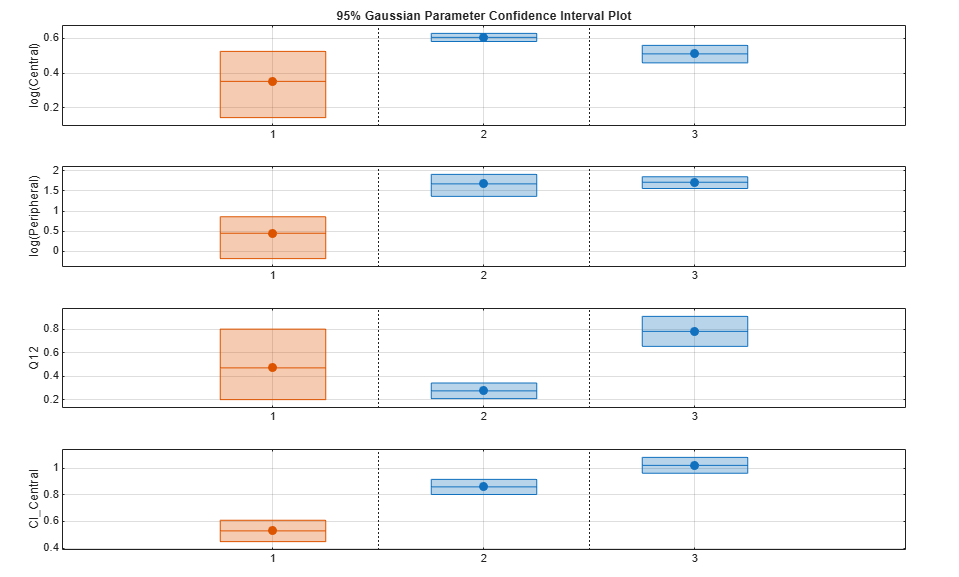Compute the confidence intervals for the pooled fit.

```ciParamPooled = sbioparameterci(pooledFit); ```

Display the confidence intervals.

```ci2table(ciParamPooled) ```
```ans = 4x7 table Group Name Estimate ConfidenceInterval Type Alpha Status ______ ______________ ________ __________________ ________ _____ ___________ pooled {'Central' } 1.6626 1.3287 1.9965 Gaussian 0.05 estimable pooled {'Peripheral'} 2.687 0.89848 4.8323 Gaussian 0.05 constrained pooled {'Q12' } 0.44956 0.11445 0.85152 Gaussian 0.05 constrained pooled {'Cl_Central'} 0.78493 0.59222 0.97764 Gaussian 0.05 estimable ```

Plot the confidence intervals. The group name is labeled as "pooled" to indicate such fit.

```plot(ciParamPooled) ```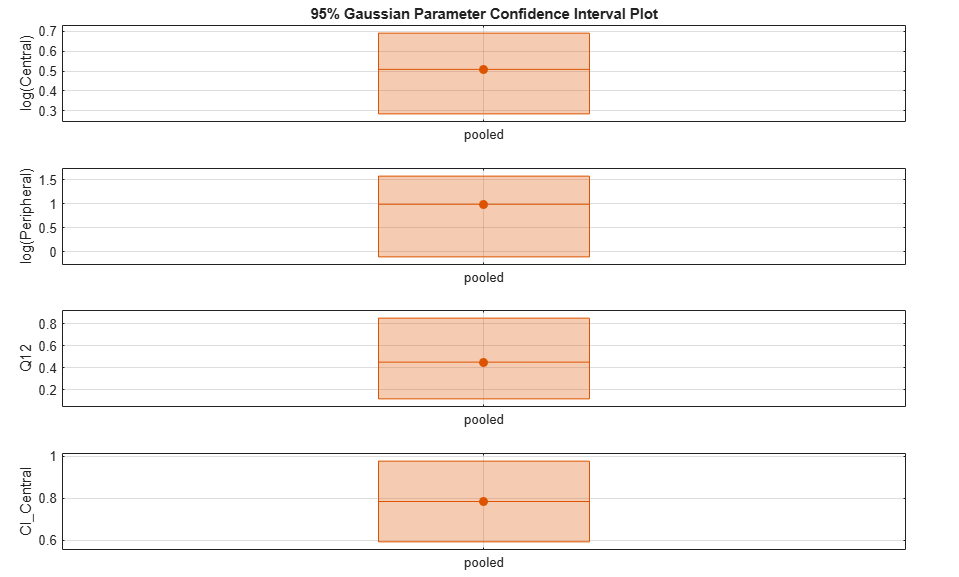Plot all the confidence interval results together. By default, the confidence interval for each parameter estimate is plotted on a separate axes. Vertical lines group confidence intervals of parameter estimates that were computed in a common fit.

```ciAll = [ciParamUnpooled;ciParamPooled]; plot(ciAll) ```You can also plot all confidence intervals in one axes grouped by parameter estimates using the 'Grouped' layout.

```plot(ciAll,'Layout','Grouped') ```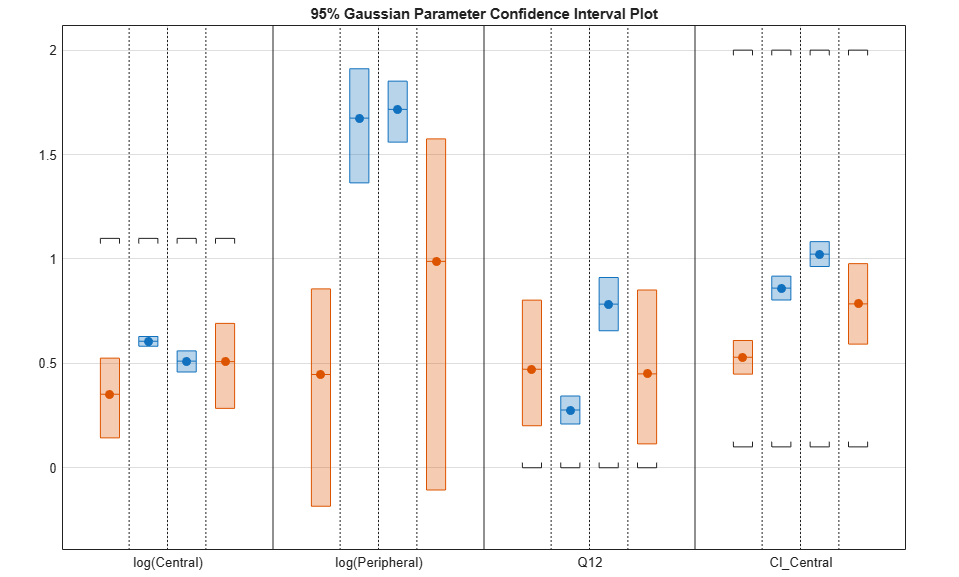In this layout, you can point to the center marker of each confidence interval to see the group name. Each estimated parameter is separated by a vertical black line. Vertical dotted lines group confidence intervals of parameter estimates that were computed in a common fit. Parameter bounds defined in the original fit are marked by square brackets. Note the different scales on the y-axis due to parameter transformations. For instance, the y-axis of `Q12` is in the linear scale, but that of `Central` is in the log scale due to its log transform.

Compute Confidence Intervals for Model Predictions

Calculate 95% confidence intervals for the model predictions, that is, simulation results using the estimated parameters.

```% For the pooled fit ciPredPooled = sbiopredictionci(pooledFit); % For the unpooled fit ciPredUnpooled = sbiopredictionci(unpooledFit); ```

Plot Confidence Intervals for Model Predictions

The confidence interval for each group is plotted in a separate column, and each response is plotted in a separate row. Confidence intervals limited by the bounds are plotted in red. Confidence intervals not limited by the bounds are plotted in blue.

```plot(ciPredPooled) ```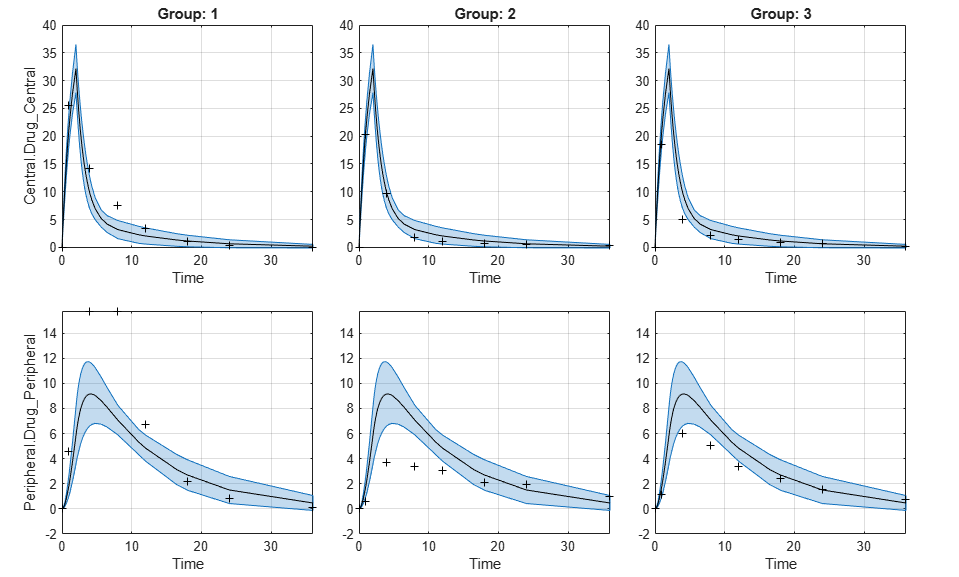```plot(ciPredUnpooled) ```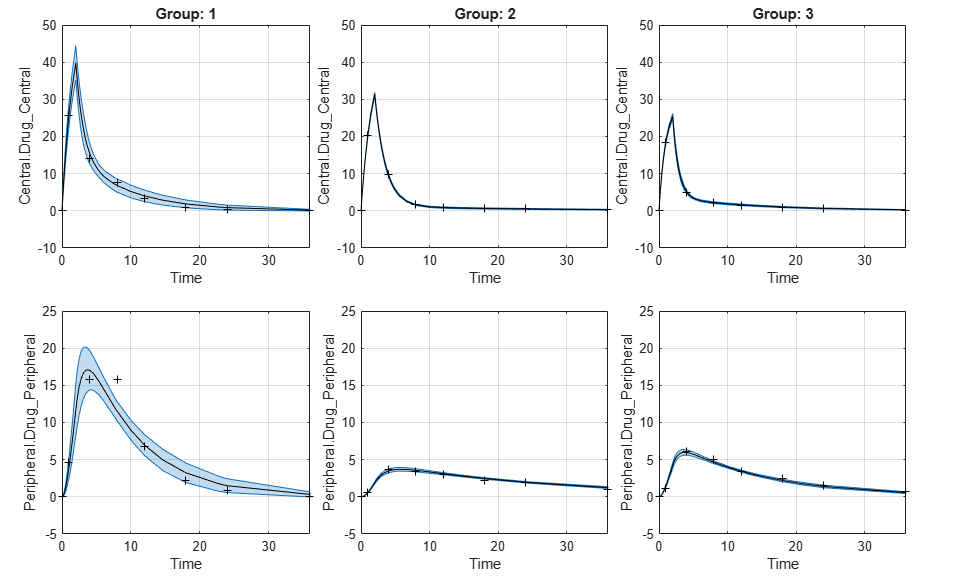expand all